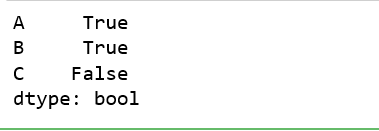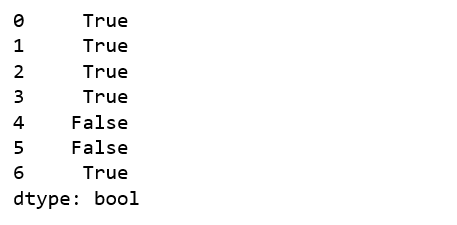# Python | Pandas Series/Dataframe.any()

• Last Updated : 16 Oct, 2018

Python is a great language for doing data analysis, primarily because of the fantastic ecosystem of data-centric python packages. Pandas is one of those packages and makes importing and analyzing data much easier.

Pandas `any()` method is applicable both on Series and Dataframe. It checks whether any value in the caller object (Dataframe or series) is not 0 and returns True for that. If all values are 0, it will return False.

Syntax: DataFrame.any(axis=0, bool_only=None, skipna=True, level=None, **kwargs)

Parameters:
axis: 0 or ‘index’ to apply method by rows and 1 or ‘columns’ to apply by columns.
bool_only: Checks for bool only series in Data frame, if none found, it will use only boolean values. This parameter is not for series since there is only one column.
skipna: Boolean value, If False, returns True for whole NaN column/row
level: int or str, specifies level in case of multilevel

Return type: Boolean series

Example #1: Index wise implementation

In this example, a sample data frame is created by passing dictionary to Pandas `DataFrame()` method. Null values are also passed to some indexes using Numpy `np.nan` to check behaviour with null values. Since in this example, the method is implemented on index, the axis parameter is kept 0 (that stands for rows).

 `# importing pandas module  ` `import` `pandas as pd  ` ` `  `# importing numpy module ` `import` `numpy as np ` ` `  `# creating dictionary ` `dic ``=` `{``'A'``: [``1``, ``2``, ``3``, ``4``, ``0``, np.nan, ``3``], ` `       ``'B'``: [``3``, ``1``, ``4``, ``5``, ``0``, np.nan, ``5``], ` `       ``'C'``: [``0``, ``0``, ``0``, ``0``, ``0``, ``0``, ``0``]} ` ` `  `# making dataframe using dictionary ` `data ``=` `pd.DataFrame(dic) ` ` `  `# calling data.any column wise ` `result ``=` `data.``any``(axis ``=` `0``) ` ` `  `# displaying result ` `result `

Output:
As shown in output, since last column is having all values equal to zero, Hence False was returned only for that column.Example #2: Column wise Implementation

In this example, a sample data frame is created by passing dictionary to Pandas `DataFrame()` method just like in above example. But instead of passing 0 to axis parameter, 1 is passed to implement for each value in every column.

 `# importing pandas module  ` `import` `pandas as pd  ` ` `  `# importing numpy module ` `import` `numpy as np ` ` `  `# creating dictionary ` `dic ``=` `{``'A'``: [``1``, ``2``, ``3``, ``4``, ``0``, np.nan, ``3``], ` `       ``'B'``: [``3``, ``1``, ``4``, ``5``, ``0``, np.nan, ``5``], ` `       ``'C'``: [``0``, ``0``, ``0``, ``0``, ``0``, ``0``, ``0``]} ` ` `  `# making dataframe using dictionary ` `data ``=` `pd.DataFrame(dic) ` ` `  `# calling data.any column wise ` `result ``=` `data.``any``(axis ``=` `1``) ` ` `  `# displaying result ` `result `

Output:
As shown in the output, False was returned for only rows where all values were 0 or NaN and 0.My Personal Notes arrow_drop_up
Recommended Articles
Page :